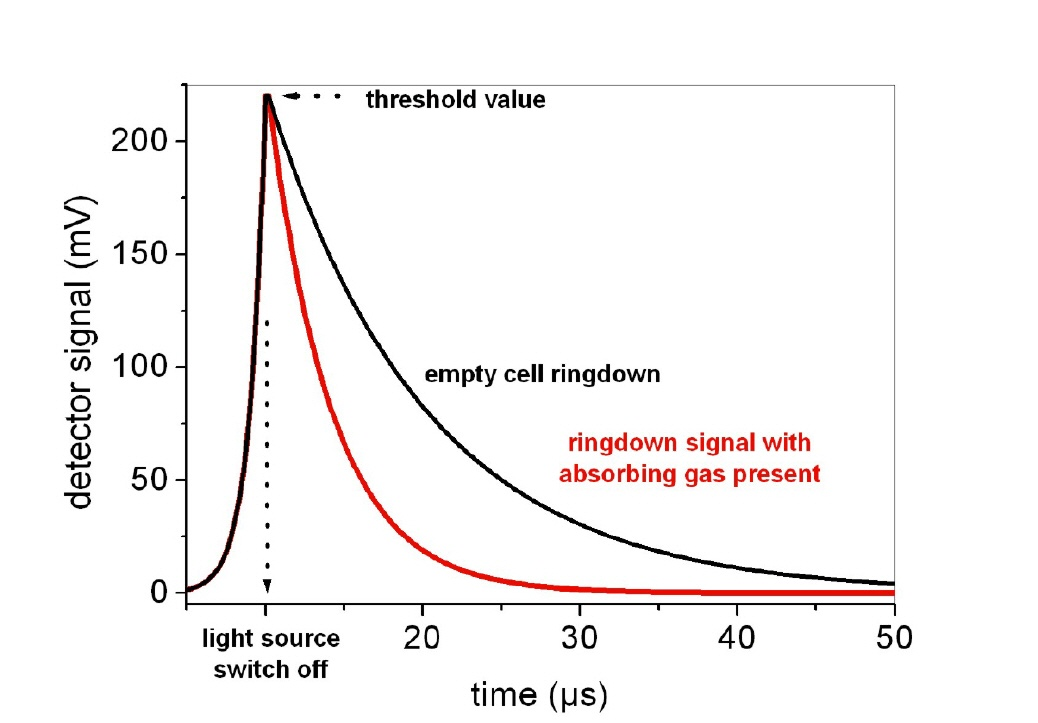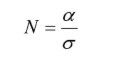# Cavity ringdown spectroscopy

• Most mentioned cavity enhanced absorption methods measure the light intensity after the resonator and derive the concentration of the absorber of interest by this signal. Cavity ring-down spectroscopy does not! Instead, the intensity decay of a “loaded” resonator is measured, and the absorber concentration is derived by the decay time. It starts with an “empty” cell (that is, there is no absorber of interest in it) which is excited (usually by a laser). When the resonator transmission reaches a certain threshold, the laser is switched off rapidly, leaving only the excited resonator (which can be pictured as a lot of photons buzzing around inside it). The subsequent decay of the transmission signal (aka the photons inside the resonator are absorbed) is then recorded, and its characteristic time constant (called ring-down time τ, typical values are around 1-100 µs) is determined. This is depicted in the next figure.fig. 1: Schematic ring-down signals with (red curve) and without (black curve) absorbing medium
• Once the “empty” cell ring-down time is measured (τ0), the sample gas with an unknown amount of the absorber is introduced into the resonator and the ring-down time measurement is repeated (yields τ). Now, since the absorber is an additional loss mechanism, the decay will be faster than before (red curve). The additional absorption coefficient can be calculated from the above mentioned ring-down times τ and τ0:
• The absorber concentration in the sample gas can be calculated if the absorption cross section σ of the absorber is known. First its number density is calculated byand after that its concentration byHere, σ is the absorption cross section of the absorber (in cm² / molec.), N is the absorber's number density (in molec. / cm³), V is Avogadro’s constant, and L is the volumina of 1 mol of gas molecules (22,4 dm³ / mol at 0°C for an ideal gas).